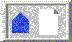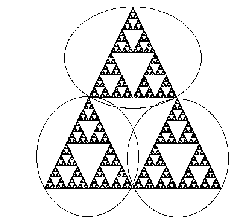Fractal Dimension (Next Section)Chaos in the Classroom (Cover Page)Playing the chaos game in (Previous Section)BU Math Home Page

# Self-similarity

One of the basic properties of fractal images is the notion of self-similarity. This idea is easy to explain using the Sierpinski triangle. Note that S may be decomposed into 3 congruent figures, each of which is exactly 1/2 the size of S! See Figure 7. That is to say, if we magnify any of the 3 pieces of S shown in Figure 7 by a factor of 2, we obtain an exact replica of S. That is, S consists of 3 self-similar copies of itself, each with magnification factor 2.Figure 7: Magnifying the Sierpinski triangle

We can look deeper into S and see further copies of S. For the Sierpinski triangle also consists of 9 self-similar copies of itself, each with magnification factor 4. Or we can chop S into 27 self-similar pieces, each with magnification factor 8. In general, we may divide S into 3^n self-similar pieces, each of which is congruent, and each of which may be maginified by a factor of 2^n to yield the entire figure. This type of self-similarity at all scales is a hallmark of the images known as fractals.Fractal Dimension (Next Section)Chaos in the Classroom (Cover Page)Playing the chaos game in (Previous Section)BU Math Home Page

Robert L. Devaney
Sun Apr 2 14:31:18 EDT 1995